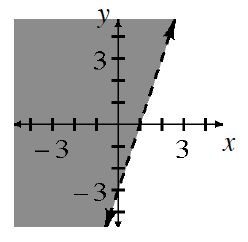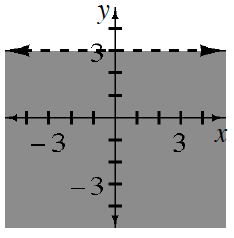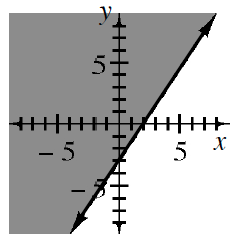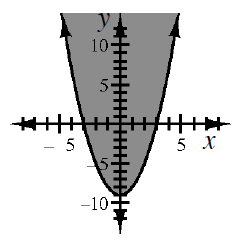### Home > CCA2 > Chapter Ch4 > Lesson 4.2.4 > Problem4-104

4-104.

Graph the solutions to each of the following inequalities on a different set of axes. Label each graph with the inequality as given and with its $y=$ form. Choose a test point and show that it gives the same result in both forms of your inequality.

1. $3x−3

$y>3x−3$1. $3>y$

$y<3$1. $3x−2y\le6$

Rewrite the inequality as an equation and solve for $y$. Graph the line.

Does the point $(0,0)$ make the original inequality true?

$\textit{y} \ge \frac{3\textit{x}}{2} -3$1. $x^2−y\le9$

Repeat the steps used in part (a): Solve for $y$; graph the parabola; test $(0,0)$; decide where to shade.

$y\ge x^2−9$